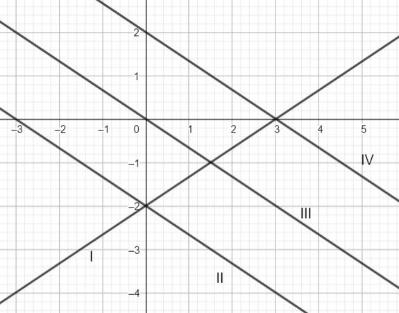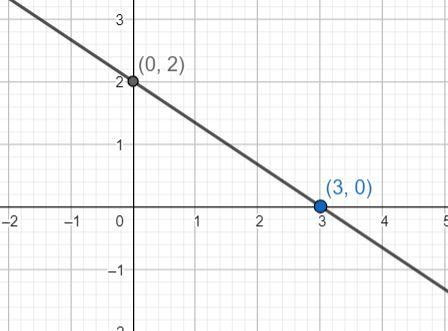QUESTION

# The graph 2x + 3y = 6 is represented by which of the following?(a). I(b). II(c). III(d). IV

Hint: Find the points through which the line 2x + 3y = 6 passes through and draw its graph and then compare with the given graphs and choose the correct option.

A line is a straight one-dimensional figure that has no thickness and extends infinitely in both directions.
A line can be represented graphically or algebraically by writing the equation of the line.
The equation involving a line is a linear equation.
We can convert the algebraic representation to graphical representations.
If the algebraic representation of the line is given, we can find the points through which the line passes through and sketch the line.
If the graphical representation is given, we take two points on the line and we can construct the algebraic linear equation.
We now have the equation of the line as 2x + 3y = 6. We can find the points and sketch the graph.
For x = 0, we have:
2(0) + 3y = 6
3y = 6
y = 2
Hence, the point is (0, 2).
Now, let y = 0, then we have:
2x + 3(0) = 6
2x = 6
x = 3
Hence, the point is (3, 0).
Hence, the line passes through the points (0, 2) and (3, 0). Then the graph is given as below:Comparing, with the options in the question, it matches with line IV.
Hence, the correct answer is option (d).

Note: We can convert the lines in the graphs to their respective equations and then also we can find the correct answer.